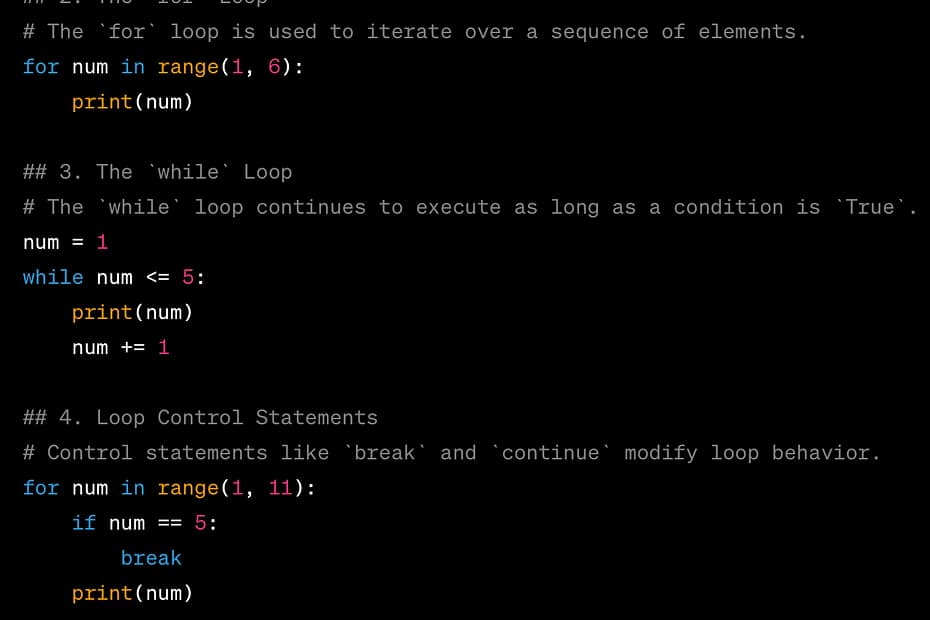# A Beginner’s Guide to Python Loops: Mastering RepetitionAre you new to the world of programming and Python? If so, you’re in the right place! One of the fundamental concepts in programming is the use of loops. Loops allow you to repeat a certain block of code multiple times, which can be incredibly powerful and time-saving. In this beginner-friendly guide, we’ll walk you through the basics of Python loops with step-by-step explanations and code snippets.

1. Introduction to Loops
2. The `for` Loop
3. The `while` Loop
4. Loop Control Statements
5. Nested Loops
6. Practical Examples
7. Conclusion

## 1. Introduction to Loops

Loops are used to execute a block of code repeatedly. They’re especially useful when you want to perform the same action on a range of values or until a certain condition is met.

## 2. The `for` Loop

The `for` loop is used to iterate over a sequence of elements, such as a list, tuple, or string. Let’s say you want to print numbers from 1 to 5:

``````for num in range(1, 6):
print(num)
``````

## 3. The `while` Loop

The `while` loop continues to execute a block of code as long as a given condition is `True`. For instance, printing numbers from 1 to 5 using a `while` loop:

``````num = 1
while num <= 5:
print(num)
num += 1
``````

## 4. Loop Control Statements

Control statements like `break` and `continue` help you modify the flow of your loops. `break` is used to exit the loop prematurely, while `continue` skips the rest of the current iteration and moves to the next one.

Example of `break`:

``````for num in range(1, 11):
if num == 5:
break
print(num)
``````

Example of `continue`:

``````for num in range(1, 6):
if num == 3:
continue
print(num)
``````

## 5. Nested Loops

You can have loops within loops, known as nested loops. This is useful when dealing with multidimensional data structures.

Example of a nested loop:

``````for i in range(1, 4):
for j in range(1, 4):
print(i, j)
``````

## 6. Practical Examples

Let’s explore a couple of practical examples.

### Example 1: Sum of Numbers

Calculate the sum of numbers from 1 to 10 using a `for` loop:

``````sum_result = 0
for num in range(1, 11):
sum_result += num
print("Sum:", sum_result)
``````

### Example 2: Factorial Calculation

Calculate the factorial of a given number using a `while` loop:

``````num = int(input("Enter a number: "))
factorial = 1
while num > 0:
factorial *= num
num -= 1
print("Factorial:", factorial)
``````

## 7. Conclusion

Congratulations! You’ve taken your first steps into the world of Python loops. You now have a solid understanding of both `for` and `while` loops, how to control their flow, and even how to use nested loops for more complex scenarios. With this knowledge, you’re well-equipped to tackle repetitive tasks and solve a wide range of programming challenges.

Remember, practice makes perfect. Experiment with loops, modify code snippets and explore various scenarios to truly solidify your understanding. Happy coding!